### Home > MC2 > Chapter 9 > Lesson 9.3.1 > Problem9-79

9-79.
1. Elyse, Paul and J'Marcus were looking at the graph below. Based on the graph, Elyse wrote the rule y = 3x + 22. Paul wrote the rule y = 22x + 3. J'Marcus wrote the rule y = 22 + 3x. Homework Help ✎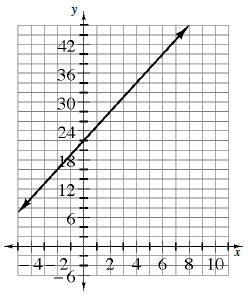1. Are the three equations equivalent, or are they different? Explain your conclusion.

2. Which equation (or equations) match the line on the graph? Justify your answer.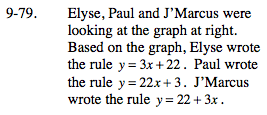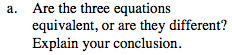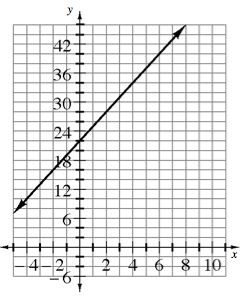Look at the different parts of each equation. Is 3x the same as 3? Is 22 the same as 22x?

Elyse and J'Marcus have the same equations because the terms can be switched around if they are related by addition.

y = 3x + 22
y = 22 + 3x

Paul's equation has a slope of 22, because 22 is the coefficient to x.
The equations that Elyse and J'Marcus wrote have a slope of only 3, so Paul's equation is different.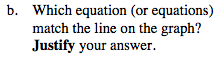$\text{What is the } \frac{\text{rise}}{\text{run}} ?$

Find the slope of the line by picking two points on the line and making a slope triangle.
In the equations above, the slope is the coefficient of x.